# Retro (Past 13 Year) JEE Main (Coordination Compounds)

## 32 Questions MCQ Test | Retro (Past 13 Year) JEE Main (Coordination Compounds)

Description
This mock test of Retro (Past 13 Year) JEE Main (Coordination Compounds) for Class 12 helps you for every Class 12 entrance exam. This contains 32 Multiple Choice Questions for Class 12 Retro (Past 13 Year) JEE Main (Coordination Compounds) (mcq) to study with solutions a complete question bank. The solved questions answers in this Retro (Past 13 Year) JEE Main (Coordination Compounds) quiz give you a good mix of easy questions and tough questions. Class 12 students definitely take this Retro (Past 13 Year) JEE Main (Coordination Compounds) exercise for a better result in the exam. You can find other Retro (Past 13 Year) JEE Main (Coordination Compounds) extra questions, long questions & short questions for Class 12 on EduRev as well by searching above.
QUESTION: 1

### The octahedral complex of a metal ion M3+ with four monodentate ligands L1 , L2, L3 and L4 absorb wavelengths in the region of red, green, yellow and blue, respectively. The increasing order of ligand strength of the four ligands is (JEE Main 2014)

Solution: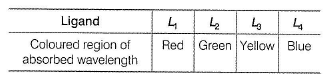Coloured regions of visible light (VIBGYOR) are arranged in the increasing order of wavelength or decreasing order of energy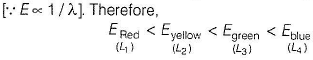This suggests that L4 will absorbs higher energy wavelength followed by L2, L3 and L1
Since, higher absorbed energy corresponds to greater extent of crystal field splitting and thus, higher field strength of the ligand Hence, the increasing order of ligand strength of the four ligands is
L1 < L3 < L2 < L4

QUESTION: 2

### Which of the following complex species is not expected to exhibit optical isomerism? (JEE Main 2013)

Solution:

Optical isomerism is exhibited by only those complexes which lacks elements of symmetry.
[Co(NH3)3CI3] shows facial as well as meridional isomerism. But both the forms contain plane of symmetry.
Thus, this complex does not exhibit optical isomerism.

QUESTION: 3

### Which amohg the following will be named as dibromidobis- (ethylenediamine) chromium (III) bromide?

Solution:

Two Br, two (en) and one Cr are parts of complex. Charge on the complex is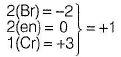Thus, complex ion is [Cr(en)2Br2]+.
Since, anion is bromide thus, complex is [Cr(en)2Br2]Br.

QUESTION: 4

Which of the following facts about the complex [Cr(NH3)6]CI3 is wrong?

(AIEEE 2011)

Solution: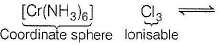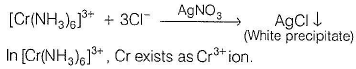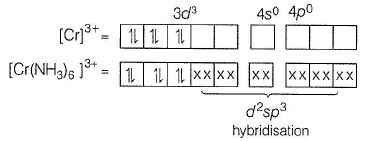The outer orbital electronic configuration of Cr is 3d3 4s°.
The orbital diagram is represented in the figure
Here,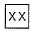indicates lone-pair of NH3 donated to Cr.
(a) d2sp3-hybridisation , octahedral. Thus, correct.
(b) There are three unpaired electrons, hence paramagnetic Thus, correct.
(c) d2sp3-inner orbital complex , thus in correct.
(d) Due to ionisable Cl- ions, white precipitate with AgNO3, thus correct.

QUESTION: 5

The magnetic moment (spin only) of [NiCI4]2- is

(AIEEE 2011)

Solution:

[NiCI4]2- ; oxidation number of Ni, x - 4 = - 2
x = + 2, Ni(28) [Ar] 3d8, 4s2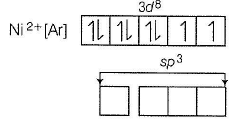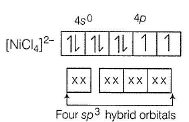sp3-hybrid orbitals, tetrahedral. Since, Cl is a weak ligand, thus unpaired electrons are not paired. Lone pairs from 4CI- are accommodated in four sp3 hybrid orbitats.
n = unpaired electron = 2, paramagnetic
Magnetic moment (spin only)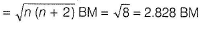QUESTION: 6

Among the ligands NH3, en, CN- and CO, the correct order of their increasing field strength is

(AIEEE 2011)

Solution:

Based on spectrochemical series, ligands arranged in increasing order of crystal field strength are as

NH3 < en < CN- < CO

QUESTION: 7

Which one of the following complex ions has geometrical isomers ?

(AIEEE 2011)

Solution: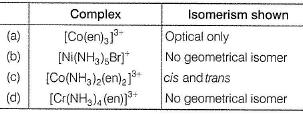QUESTION: 8

Which one of the following has an optical isomer? (en = ethylenediamine)

(AIEEE 2010)

Solution:

Complex [Co(en)3]3+ has no plane of symmetry and centre of symmetry. That’s why, it is optically active.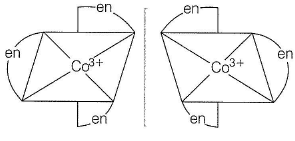QUESTION: 9

Which of the following has an optical isomer?

Solution: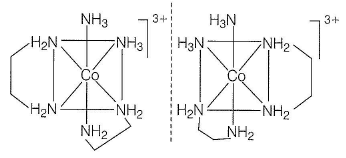QUESTION: 10

Which of the following pairs represents linkage isomers?

(AIEEE 2009)

Solution:

Linkage isomers are caused due to the presence of ambidentate ligands.
[Pd(PPh3)2(NCS)2] and [Pd(PPh3)2(SCN)2] are linkage isomers due to SCN, an ambidentate ligand.
Thus, SCN can linked to Pd by both N as well as S through their lone pairs.

QUESTION: 11

The coordination number and the oxidation state of the element 'E’ in the complex [E(en)2(C2O4)] NO2 (where, (en) is ethylene diamine) respectively are

(AIEEE 2008)

Solution:

en,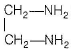is bidentate ligand.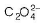is also bidentate ligand.
Hence, coordination number of the element E in the given complex comes out to be 6.
The complex can be ionised as
[E(en)2(C2O4)] NO2 →[E(en)2 (C2O4)]+ NO2-
Oxidation number, x + 0 + (- 2) = + 1
x = 3

QUESTION: 12

In which of the following octahedral complexes of Co (atomic number 27), will the magnitude of Δ be the highest?

(AIEEE 2008)

Solution:

CFSE (crystal field splitting energy) for octahedral complex, Δo depends on the strength of negative ligand, Spectrochemically, it has been found that the strength of splitting is as follows :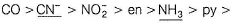NCS- > H2O > O2- > ox2- > OH- > F- > Cl- > SCN- > S2- > Br- > l-

QUESTION: 13

Which one of the following has a square planar geometry?

(Atomic number, Co = 27, Ni = 28, Fe = 26, Pt =78

(AIEEE 2007)

Solution:

Cl- is aweak ligand but Cl- cause the pairing of electrons with large Pt2+ and consequently give dsp2 hybridisation and square planar geometry.

QUESTION: 14

In Fe(CO)5, the Fe—CO bond possesses

Solution:

Fe(CO)5 is an organometallic coordinated compound in which electrons from d-orbital of Fe are partially transferred to antibonding molecular orbitals (π) of CO. This type of bonding is called π-back bonding.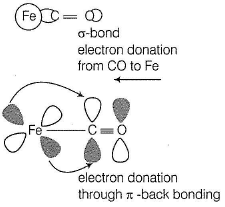Thus, Fe(CO)5 possesses both σ and π-character.

QUESTION: 15

The IUPAC name for the complex [Co (NO2) (NH3)5] Cl2 is

(AIEEE 2006)

Solution:

The IUPAC name for the complex
[Co(NO2)(NH3)5]CI2
Pentammine nitrito-N-cobalt (III) chloride

QUESTION: 16

Nickel (Z = 28) combines with a uninegative monodentate ligand X- to form a paramagnetic complex [NiX4]2-. The number of unpaired electron(s) in the nickel and geometry of this complex ion, respectively are

(AIEEE 2006)

Solution:

28Ni = [Ar] 4s2, 3d8
Ni2+ = [Ar] 3d8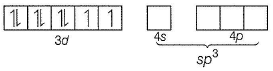Nickel has two unpaired electrons and geometry is tetrahedral due to sp3 hybridisation.

QUESTION: 17

The value of the spin only magnetic moment for one of the following configurations is 2.84 BM. The correct one is

(AIEEE 2005)

Solution:

(a) d5 in strong field
n = unpaired electron = 1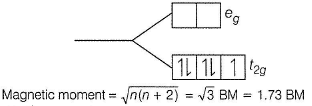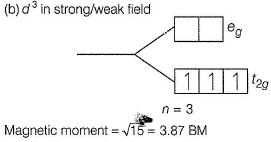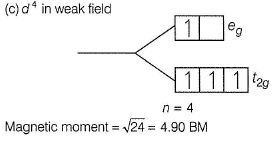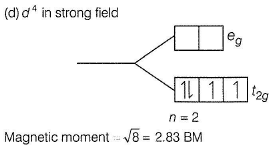QUESTION: 18

Which of the following factors may be regarded as the main cause of lanthanide contraction?

(AIEEE 2005)

Solution:

Lanthanide contraction is due to poor shielding of one of 4f electron by another in the subshell.

QUESTION: 19

The lanthanide contraction is responsible for the fact that

(AIEEE 2005)

Solution:

Due to lanthanoid contraction, the atomic radii of the elements belonging to the same group of the 2nd and 3rd transition series become same. Thus, 40Zr (160 pm) and 72Hf (159 pm) have almost same radii.

QUESTION: 20

The IUPAC name of the coordination compound K3[Fe(CN)6] is

Solution:

K3[Fe(CN)6]
Cation Anion Oxidation state of Fe in anion = + 3
Thus, it is potassium hexacyanoferrate (III).

QUESTION: 21

Which one of the following cyano complexes would exhibit the lowest value of paramagnetic behaviour?

(Atomic number of Cr = 24, Mn = 25, Fe = 26, Co = 27)

(AIEEE 2005)

Solution: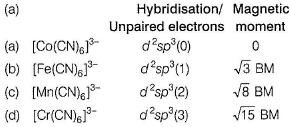Thus, least paramagnetism is (a).

QUESTION: 22

Which of the following compounds shows optical isomerism?

(AIEEE 2005)

Solution: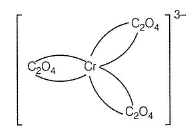Mirror image is not superimposable, hence optical isomerism is possible.

QUESTION: 23

The coordination number of a central metal atom in a com plex is determ ined by

(AIEEE 2004)

Solution:

Coordination number is the maximum covalency shown by a metal or metal ion. It is the maximum number of ligands attached to metal by sigma bonds or coordinate bonds.

QUESTION: 24

Which one of the following has largest number of isomers ?

(AIEEE 2004)

Solution:

All the given compounds exhibit geometrical (cis and trans isomers) but only cis isomer of [Co (en)2CI2]+ possess optical isomers (d and /) due to the presence of symmetrical didentate, en [ethylenediamine],

QUESTION: 25

The correct order of magnetic moments (spin only values in BM) among the following is

(atomic number of Mn = 25, Fe = 26, Co = 27)

(AIEEE 2004)

Solution:

Number of unpaired electrons in [Fe(CN)6]4- is zero.
Thus, magnetic moment =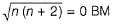(n = unpaired electrons)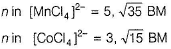QUESTION: 26

Which one of the following complexes is an outer orbital complex?

(Atomic number of Mn = 25, Fe = 26, Co = 27, Ni = 28)

(AIEEE 2004)

Solution: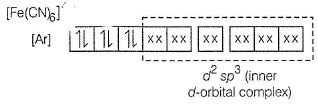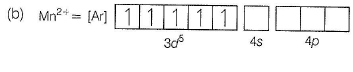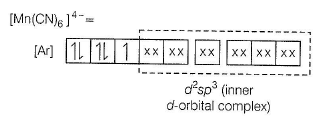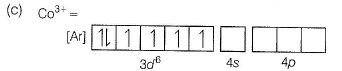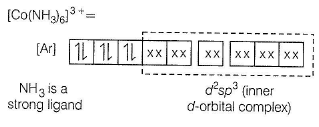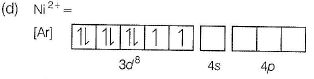In this case also, NH3 is a strong ligand but electrons remain unpaired, since only one orbital is left vacant in 3d. Thus, [Ni(NH3)6]2+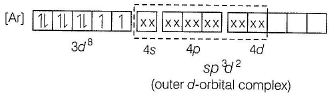QUESTION: 27

Ammonia forms the complex ion [Cu(NFl3)4]2+ with copper ions in the alkaline solutions but not in acidic solutions. What is the reason for it?

(AIEEE 2003)

Solution:

In acidic solutions, N-atoms of NH3 donates their lone pair electrons to protons forming NH4+ ions.
Therefore, lone pair electrons of NH3 are not available for forming the complex with copper ions.

QUESTION: 28

One mole of the complex compound Co(NFl3)5Cl3 gives 3 moles of ions on dissolution in water. One mole of the same complex reacts with tw o moles of AgNO3 solution to yield two moles of AgCI(s). The structure of the complex is

(AIEEE 2003)

Solution:

The oxidation state of nickel is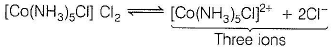QUESTION: 29

In the coordination compound, K4[Ni(CN)4]the oxidation state of nickel is

(AIEEE 2003)

Solution:

The oxidation state of nickel is
K4[Ni(CN)4] → 4K+ + [Ni(CN)4]4-
[Ni(CN)4]4- , x + (4 x - 1) = - 4
or x - 4 = - 4 or x = 0

QUESTION: 30

Type of isom erism shown by [Cr(NH3)5NO2] Cl2 is

(AIEEE 2002)

Solution:

[Cr(NH3)5NO2]Cl2 (pentam m inenitritochromium (III) chloride) exhibits linkage isomerism as—NO2 is am bid entate ligand. Isomer is [Cr(NH3)5ONO] Cl2.

QUESTION: 31

A square planar complex is formed by the hybridisation of which of the following atomic orbitals?

(AIEEE 2002)

Solution:

A square planar geometry is the result of dsp2 hybridisation where innerd subshell (dx2-yorbital) participates.

QUESTION: 32

The most stable ion is

(AIEEE 2002)

Solution:

Stability of complex increases with increase in charge on the central metal ion and with increase in basic strength of ligand.
In [Fe(CN)6]3-, oxidation state of Fe is +3 and basicity of CN- is higher than OH-, Cl- and H2O.